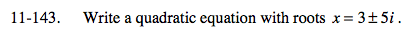### Home > A2C > Chapter Ch11 > Lesson 11.3.2 > Problem11-143

11-143.

Write a quadratic equation with roots x = 3 ± 5i. Homework Help ✎If the roots are x = ± 5i, then the factors must be (x − (3 + 5i)) and (x − (3 − 5i)).

Use the factors to write an equation.
(x − 3 + 5i)(x − 3 − 5i) = 0

Multiply.
(x − 3)2 − 25i 2 = 0

x2 − 6x + 34 = 0